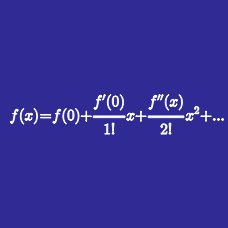Calculus

# Taylor Series: Level 3 Challenges

Calculate the sum of series below. $\frac{\pi^2}{3!}-\frac{\pi^4}{5!}+\frac{\pi^6}{7!}-\frac{\pi^8}{9!}+\cdots$

$1 + \frac{1+2}{2!} + \frac{1+2+2^2}{3!} + \frac{1+2+2^2+2^3}{4!} + \ldots$

Find the coefficient of $x^2$ in the Maclaurin series expansion for $\large (1+e^{x})^{10}.$

Evaluate the 25th derivative of $x^3\sin(x^2) \text{ at } x = 0.$

Hint: Start with the Maclaurin series for $\sin(x)$ to obtain a series for $\sin(x^2)$ and then for $x^3 \sin(x^2).$

$\large\dfrac1{1\cdot2}+\dfrac1{3\cdot4}+\dfrac1{5\cdot6}+\dfrac1{7\cdot8}+\ldots$

Let $S$ denote the value of series above. Find the value of $e^S$.

×